# Molecular geometry

﻿
Molecular geometry

Molecular geometry or molecular structure is the three-dimensional arrangement of the atoms that constitute a molecule. It determines several properties of a substance including its reactivity, polarity, phase of matter, color, magnetism, and biological activity.

## Molecular geometry determination

The molecular geometry can be determined by various spectroscopic methods and diffraction methods. IR, microwave and Raman spectroscopy can give information about the molecule geometry from the details of the vibrational and rotational absorbances detected by these techniques. X-ray crystallography, neutron diffraction and electron diffraction can give molecular structure for crystalline solids based on the distance between nuclei and concentration of electron density. Gas electron diffraction can be used for small molecules in the gas phase. NMR and FRET methods can be used to determine complementary information including relative distances,  dihedral angles,   angles, and connectivity. Molecular geometries are best determined at low temperature because at higher temperatures the molecular structure is averaged over more accessible geometries (see next section). Larger molecules often exist in multiple stable geometries (conformational isomerism) that are close in energy on the potential energy surface. Geometries can also be computed by ab initio quantum chemistry methods to high accuracy. The molecular geometry can be different as a solid, in solution, and as a gas.

The position of each atom is determined by the nature of the chemical bonds by which it is connected to its neighboring atoms. The molecular geometry can be described by the positions of these atoms in space, evoking bond lengths of two joined atoms, bond angles of three connected atoms, and torsion angles (dihedral angles) of three consecutive bonds.

## The influence of thermal excitation

Since the motions of the atoms in a molecule are determined by quantum mechanics, one must define “motion” in a quantum mechanical way. The overall (external) quantum mechanical motions translation and rotation hardly change the geometry of the molecule. (To some extent rotation influences the geometry via Coriolis forces and centrifugal distortion, but this is negligible for the present discussion.) A third type of motion is vibration, which is the internal motion of the atoms in a molecule. The molecular vibrations are harmonic (at least to good approximation), which means that the atoms oscillate about their equilibrium, even at the absolute zero of temperature. At absolute zero all atoms are in their vibrational ground state and show zero point quantum mechanical motion, that is, the wavefunction of a single vibrational mode is not a sharp peak, but an exponential of finite width. At higher temperatures the vibrational modes may be thermally excited (in a classical interpretation one expresses this by stating that “the molecules will vibrate faster”), but they oscillate still around the recognizable geometry of the molecule.

To get a feeling for the probability that the vibration of molecule may be thermally excited, we inspect the Boltzmann factor$\exp\left( -\frac{\Delta E}{kT} \right)$, where ΔE is the excitation energy of the vibrational mode, k the Boltzmann constant and T the absolute temperature. At 298K (25 °C), typical values for the Boltzmann factor are: 0.089 for ΔE = 500 cm−1 ; ΔE = 0.008 for 1000 cm−1 ; 7 10−4 for ΔE = 1500 cm−1. That is, if the excitation energy is 500 cm−1, then about 9 percent of the molecules are thermally excited at room temperature. The lowest excitation vibrational energy in water is the bending mode (about 1600 cm−1). Thus, at room temperature less than 0.07 percent of all the molecules of a given amount of water will vibrate faster than at absolute zero.

As stated above, rotation hardly influences the molecular geometry. But, as a quantum mechanical motion, it is thermally excited at relatively (as compared to vibration) low temperatures. From a classical point of view it can be stated that more molecules rotate faster at higher temperatures, i.e., they have larger angular velocity and angular momentum. In quantum mechanically language: more eigenstates of higher angular momentum become thermally populated with rising temperatures. Typical rotational excitation energies are on the order of a few cm−1.

The results of many spectroscopic experiments are broadened because they involve an averaging over rotational states. It is often difficult to extract geometries from spectra at high temperatures, because the number of rotational states probed in the experimental averaging increases with increasing temperature. Thus, many spectroscopic observations can only be expected to yield reliable molecular geometries at temperatures close to absolute zero, because at higher temperatures too many higher rotational states are thermally populated.

## Bonding

Molecules, by definition, are most often held together with covalent bonds involving single, double, and/or triple bonds, where a "bond" is a shared pair of electrons (the other method of bonding between atoms is called ionic bonding and involves a positive cation and a negative anion).

Molecular geometries can be specified in terms of bond lengths, bond angles and torsional angles. The bond length is defined to be the average distance between the centers of two atoms bonded together in any given molecule. A bond angle is the angle formed between three atoms across at least two bonds. For four atoms bonded together in a chain, the torsional angle is the angle between the plane formed by the first three atoms and the plane formed by the last three atoms.

There exists a mathematical relationship among the bond angles for one central atom and four peripheral atoms (labeled 1 through 4) expressed by the following determinant. This constraint removes one degree of freedom from the choices of (originally) six free bond angles to leave only five choices of bond angles. (Note that the angles θ11, θ22, θ33, and θ44 are always zero.)$0 = \begin{vmatrix} \cos \theta_{11} & \cos \theta_{12} & \cos \theta_{13} & \cos \theta_{14} \\ \cos \theta_{21} & \cos \theta_{22} & \cos \theta_{23} & \cos \theta_{24} \\ \cos \theta_{31} & \cos \theta_{32} & \cos \theta_{33} & \cos \theta_{34} \\ \cos \theta_{41} & \cos \theta_{42} & \cos \theta_{43} & \cos \theta_{44} \end{vmatrix}$

Molecular geometry is determined by the quantum mechanical behavior of the electrons. Using the valence bond approximation this can be understood by the type of bonds between the atoms that make up the molecule. When atoms interact to form a chemical bond, the atomic orbitals are said to mix in a process called orbital hybridisation. The two most common types of bonds are Sigma bonds and Pi bonds. The geometry can also be understood by molecular orbital theory where the electrons are delocalised.

An understanding of the wavelike behavior of electrons in atoms and molecules is the subject of quantum chemistry.

## Isomers

Isomers are types of molecules that share a chemical formula but have different geometries, resulting in very different properties:

• A pure substance is composed of only one type of isomer of a molecule (all have the same geometrical structure).
• Structural isomers have the same chemical formula but different physical arrangements, often forming alternate molecular geometries with very different properties. The atoms are not bonded (connected) together in the same orders.
• Functional isomers are special kinds of structural isomers, where certain groups of atoms exhibit a special kind of behavior, such as an ether or an alcohol.
• Stereoisomers may have many similar physicochemical properties (melting point, boiling point) and at the same time very different biochemical activities. This is because they exhibit a handedness that is commonly found in living systems. One manifestation of this chirality or handedness is that they have the ability to rotate polarized light in different directions.

## Types of molecular structure

Main article: VSEPR theory

There are six basic shape types for molecules

• Linear: In a linear model, atoms are connected in a straight line. The bond angles are set at 180°. A bond angle is very simply the geometric angle between two adjacent bonds. For example, carbon dioxide and nitric oxide have a linear molecular shape.
• Trigonal planar: Just from its name, it can easily be said that molecules with the trigonal planar shape are somewhat triangular and in one plane (meaning a flat surface). Consequently, the bond angles are set at 120°. An example of this is boron trifluoride.
• Bent: Bent or angular molecules have a non-linear shape. A good example is water, or H2O, which has an angle of about 105o A water molecule has two pairs of bonded electrons and two unshared lone pairs.
• Tetrahedral: Tetra- signifies four, and -hedral relates to a surface, so tetrahedral almost literally means "four surfaces." This is when there are four bonds all on one central atom, with no extra unshared electron pairs. In accordance with the VSEPR (valence-shell electron pair repulsion theory), the bond angles between the electron bonds are arccos(−1/3) = 109.47°. An example of a tetrahedral molecule is methane (CH4).
• Octahedral: Octa- signifies eight, and -hedral relates to a surface, so octahedral almost literally means "eight surfaces." The bond angle is 90 degrees. An example of an octahedral molecule is sulfur hexafluoride (SF6).
• Pyramidal: Pyramidal-shaped molecules have pyramid-like shapes. Unlike the linear and trigonal planar shapes but similar to the tetrahedral orientation, pyramidal shapes requires three dimensions in order to fully separate the electrons. Here, there are only three pairs of bonded electrons, leaving one unshared lone pair. Lone pair - bond pair repulsions change the angle from the tetrahedral angle to a slightly lower value. An example is NH3 (ammonia).

### VSEPR table

The bond angles in the table below are ideal angles from the simple VSEPR theory, followed by the actual angle for the example given in the following column where this differs. For many cases, such as trigonal pyramidal and bent, the actual angle for the example differs from the ideal angle, but all examples differ by different amounts. For example, the angle in H2S (92°) differs from the tetrahedral angle by much more than the angle for H2O (104.5°) does.

Bonding electron pairs Lone pairs Electron domains (Steric #) Shape Ideal bond angle (example's bond angle) Example Image
2
0
2
linear
180°
CO2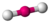3
0
3
trigonal planar
120°
BF3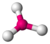2
1
3
bent
120° (119°)
SO2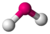4
0
4
tetrahedral
109.5°
CH4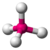3
1
4
trigonal pyramidal
107.5°
NH3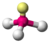2
2
4
angular
104.5°
H2O5
0
5
trigonal bipyramidal
90°, 120°, 180°
PCl5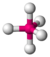4
1
5
seesaw
180°, 120°, 90° (173.1°, 101.6°)
SF4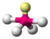3
2
5
T-shaped
90°, 180° (87.5°, < 180°)
ClF3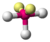2
3
5
linear
180°
XeF26
0
6
octahedral
90°, 180°
SF6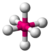5
1
6
square pyramidal
90° (84.8°), 180°
BrF5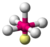4
2
6
square planar
90° 180°
XeF4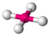7
0
7
pentagonal bipyramidal
90°, 72°
IF7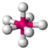## 3D Representations

• Line or stick - atomic nuclei are not represented, just the bonds as sticks or lines. As in 2D molecular structures of this type, atoms are implied at each vertex.
• Ball and stick - atomic nuclei are represented by spheres (balls) and the bonds as sticks
• Spacefilling models or CPK models (also an atomic coloring scheme in representations) - the molecule is represented by overlapping spheres representing the atoms
• Cartoon - a representation used for proteins where loops, beta sheets, alpha helices are represented diagrammatically and no atoms or bonds are represented explicitly just the protein backbone as a smooth pipe

The more the amount of lone pairs contained in an molecule the smaller the angles between the atoms of that molecule. The Valence Shell Electron-Pair Repulsion theory VSEPR predicts that lone pairs repel eachother thus pushing the different atoms away from them.

Wikimedia Foundation. 2010.

### Look at other dictionaries:

• Octahedral molecular geometry — Idealized structure of a compound with octahedral coordination geometry. In chemistry, octahedral molecular geometry describes the shape of compounds where in six atoms or groups of atoms or ligands are symmetrically arranged around a central… …   Wikipedia

• Square pyramidal molecular geometry — In molecular geometry, square based pyramidal geometry describes the shape of certain compounds with the formula ML5 where L is a ligand. If the ligand atoms were connected, the resulting shape would be that of a pyramid with a square base. The… …   Wikipedia

• Trigonal pyramidal molecular geometry — Infobox molecular geometry Symmetry group= C3v Electron direction=4 Atom direction=3 Bond angle …   Wikipedia

• Square planar molecular geometry — The square planar molecular geometry in chemistry describes the stereochemistry (spatial arrangement of atoms) that is adopted by certain chemical compounds. As the name suggests, molecules of this geometry have their atoms positioned at the… …   Wikipedia

• Seesaw molecular geometry — Infobox molecular geometry Symmetry group= C2V Electron direction=5 Atom direction=4 Bond angle=90°, 120°, 180° mu= >0 Examples= SF4 Seesaw is a type of molecular geometry where the central atom has one lone pair of electrons, and there are four… …   Wikipedia

• Trigonal bipyramidal molecular geometry — Infobox molecular geometry Symmetry group= D3h Electron direction=5 Atom direction=5 Bond angle=90°, 120° mu= 0 Examples=PF5 In chemistry a trigonal bipyramid formation is a molecular geometry with one atom at the center and 5 more atoms at the… …   Wikipedia

• Trigonal planar molecular geometry — In chemistry, trigonal planar is a molecular geometry with one atom at the center and three atoms at the corners of a triangle all in one planeFact|date=January 2008. In an ideal trigonal planar species, all three ligands are identical and all… …   Wikipedia

• Tetrahedral molecular geometry — In a Tetrahedral molecular geometry a central atom is located at the center with four substituents that are located at the corners of a tetrahedron. The bond angles are cos−1(−1/3) ≈ 109.5° when all four substituents are the same, as in CH4. This …   Wikipedia

• T-shaped molecular geometry — In chemistry, T shaped molecular geometry describes a somewhat unusual geometry adopted by compounds where a central atom has three ligands. Ordinarily, three coordinated compounds minimize the steric repulsion between the ligands by adopting… …   Wikipedia

• Bent molecular geometry — In chemistry, the term bent can be applied to certain molecules to describe their molecular geometry. H2O is an example of a bent molecule. The bond angle between the two hydrogen atoms is approximately 104.45°. [G. L. Miessler and D. A. Tarr… …   Wikipedia